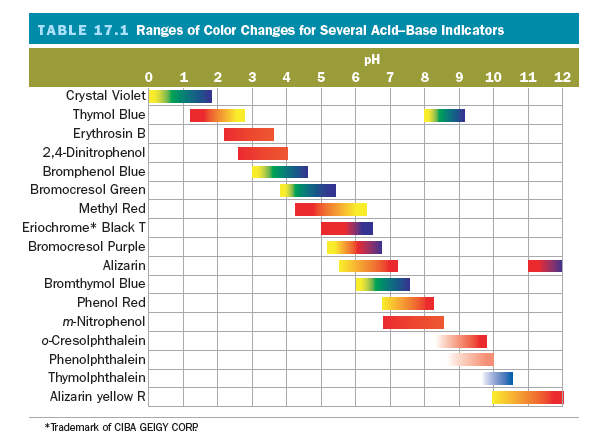# Problem: A 0.70 g sample of a weak monoprotic acid was dissolved in 150 mL of water and titrated with a 0.055 M solution of KOH at 25°C. At the half-equivalence point, after addition of 25.5 mL of the KOH solution, the pH was 3.8.What indicator would you use for the above titration? What color change would occur at the end point of the titration?A. phenol redB. phenolphthaleinC. bromcresol purpleD.  bromphenol blueE. methyl red

###### FREE Expert Solution

We are being asked to determine a suitable indicator for the titration of a weak monoprotic acid (HA) with a 0.055 M solution of KOH.

We will calculate the pH of the solution at the equivalence point using the following steps:

Step 1. Write the chemical equation for the reaction between HA and KOH.
Step 2. Calculate the initial amounts of HA and KOH in moles before the reaction happens.
Step 3. Construct an ICF Chart.
Step 4. Calculate the new concentration of the species left in the solution.
Step 5. Calculate pH at Equivalence Point.

Step 6. Determine a suitable indicator.

Step 1. Write the chemical equation for the reaction between HA and KOH.

80% (148 ratings)###### Problem Details

A 0.70 g sample of a weak monoprotic acid was dissolved in 150 mL of water and titrated with a 0.055 M solution of KOH at 25°C. At the half-equivalence point, after addition of 25.5 mL of the KOH solution, the pH was 3.8.

What indicator would you use for the above titration? What color change would occur at the end point of the titration?

A. phenol red

B. phenolphthalein

C. bromcresol purple

D.  bromphenol blue

E. methyl red